Next: 3.6.2 The Relaxation Time Up: 3.6 Energy Relaxation Time Previous: 3.6 Energy Relaxation Time

Subsections

## 3.6.1 Methodology

Depending on the semiconductor material under investigation, different results are available from Monte-Carlo simulation. Two methods - direct and indirect - are used to obtain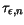.

### 3.6.1.1 The Direct Method

For Si, Ge and GaAs, the dependence of the electron energy relaxation time and the average electron energy,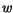, on the electric field are available in . The average energy is approximated by the thermal energy, with the kinetic term being neglected: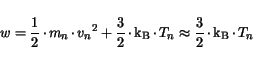(3.136)

where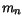,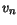, and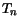are the electron mass, velocity and temperature, respectively. This approximation, together with the interpolation of the Monte-Carlo simulation results for different electric fields allows to obtain directlyas a function of the electron temperature at different lattice temperatures. This procedure is called direct method.

### 3.6.1.2 The Indirect Method

In the case of binary and ternary III-V compounds, such as InAs, AlAs, In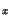Ga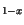As, and AlGaAs, the dependence ofon the electric field is not available. In this caseis calculated in an indirect way, using the dependence of the electron velocity on the electric field from . The local energy balance equation  is obtained by neglecting the energy flux:(3.137)

where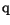is the electron charge,the lattice temperature, and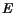is the electric field. Using (3.136) and the dependences of the average electron energy and the electron velocity on the electric field,is extracted. This procedure is called indirect method.

Fig. 3.39 showsfor GaAs as a function of the electron temperature at=300 K, as it results from both the direct and indirect methods. The correct values are extracted by the direct method as it is based on less approximations. It turns out thatis overestimated by using (3.137) in the indirect method. Nevertheless, the saturation value ofat high electron temperatures,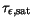, and the location of the peak,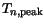, are independent of the methodology used. This criteria are used for correct estimation ofin materials where only the indirect method can be applied.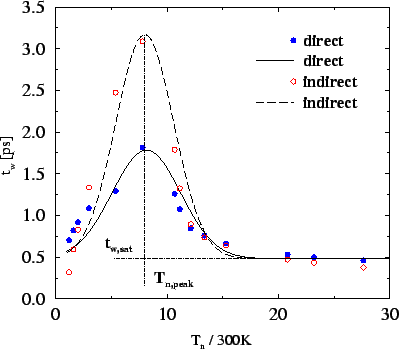Next: 3.6.2 The Relaxation Time Up: 3.6 Energy Relaxation Time Previous: 3.6 Energy Relaxation Time
Vassil Palankovski
2001-02-28